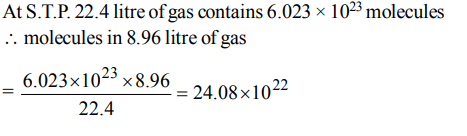## Some Basic Concepts of Chemistry Questions and Answers Part-9

1. On analysis a certain compound was found to contain iodine and oxygen in the ratio of 254 gm of iodine (atomic mass 127) and 80 gm oxygen (at mass = 16). What is the formula of the compound.
a) IO
b) $I_{2}O$
c) $I_{5}O_{3}$
d) $I_{2}O_{5}$

Explanation:2. Two oxides of a metal contain 50% and 40% metal (M)respectively. If the formula of first oxide is $MO_{2}$  the formula of second oxide will be
a) $MO_{2}$
b) $MO_{3}$
c) $M_{2}O$
d) $M_{2}O_{5}$

Explanation: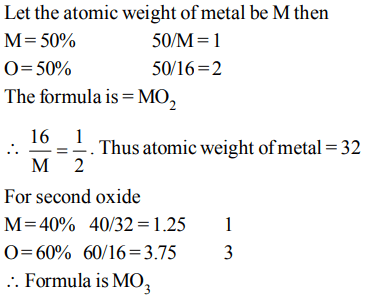3. The ratio of the molar amounts of H2S needed to precipitate the metal ions form 20 ml each of  $1 M Ca\left(NO_{3}\right)_{2} and 0.5M CuSO_{4}$     is
a) 1 : 1
b) 2 : 1
c) 1 : 2
d) indefinite

Explanation: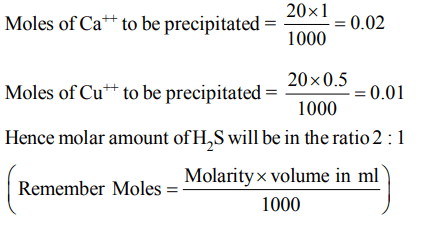4. 12 gm of Mg (atomic mass 24) will react completely with hydrochloric acid to give
a) One mol of  $H_{2}$
b) 1/2 mol of  $H_{2}$
c) 2/3 mol of  $O_{2}$
d) both 1/2 mol of  $H_{2}$  and 1/2 mol of  $O_{2}$

Explanation: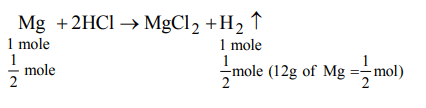5. The total number of protons in 10 gm of calcium carbonate is $\left(N_{o}=6.023×10^{23}\right)$
a) $1.5057 ×10^{24}$
b) $2.0478 ×10^{24}$
c) $3.0115 ×10^{24}$
d) $14.0956 ×10^{24}$

Explanation:6. 2.76 gm of silver carbonate (at mass of Ag 108) on being heated strongly yield a residue weighing
a) 2.16 gm
b) 2.48 gm
c) 2.32 gm
d) 2.64 gm

Explanation: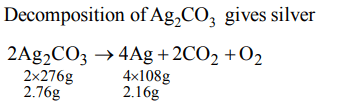7. If 0.5 mol of$BaCI_{2}$  is mixed with  0.2 mol of $Na_{3}PO_{4}$   then maximum number of moles of  $Ba_{3}\left(PO_{4}\right)_{2}$  that can be formed is
a) 0.7
b) 0.5
c) 0.3
d) 0.1

Explanation:8. 1.12 ml of a gas is produced at S.T.P. by the action of 4.12 mg of alcohol ROH with methyl magnesium Iodide. The molecular mass of alcohol is
a) 16.0
b) 41.2
c) 82.46
d) 156.0

Explanation:9. An unsaturated hydrocarbon weighing 1.68 gm has volume of 488 ml at S.T.P. If it contains 14% of hydrogen, then the family to which the hydrocarbon belongs is
a) alkane
b) alkene
c) alkyl
d) benzenea) $6.023 ×10^{23}$
b) $12.04 ×10^{23}$
c) $18.06 ×10^{23}$
d) $24.08 ×10^{22}$